A current wire is hidden in a wall. Its position can be located with the help of a

1.  Moving coil galvanometer

2.  Voltmeter

3.  Hotwire ammeter

4.  Magnetic needle

Concept Questions :-

Magnetic field due to various cases
High Yielding Test Series + Question Bank - NEET 2020

Difficulty Level:

Which of the following graph correctly represents the variation of magnetic field induction with distance due to a thin wire carrying current?

1.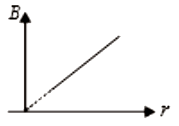2.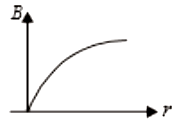3.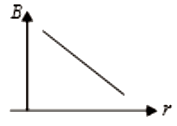4.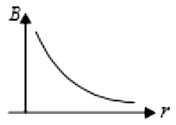Concept Questions :-

Magnetic field due to various cases
High Yielding Test Series + Question Bank - NEET 2020

Difficulty Level:

When an electron enters perpendicularly in a magnetic field with velocity v, time period of its revolution is T. If it enters in the same magnetic field with a velocity 2v, then its time period will be:

1.  2T

2.  4T

3.  $\frac{\mathrm{T}}{2}$

4.   T

Concept Questions :-

Lorentz force
High Yielding Test Series + Question Bank - NEET 2020

Difficulty Level:

1.  R

2.  2R

3.  3R

4.  $\frac{\mathrm{R}}{2}$

Concept Questions :-

Magnetic field due to various cases
High Yielding Test Series + Question Bank - NEET 2020

Difficulty Level:

When positively charged particle falling vertically downward, then the particle deflected due to earth magnetic field towards

(1) east

(2) west

(3) north

(4) south

Concept Questions :-

Lorentz force
High Yielding Test Series + Question Bank - NEET 2020

Difficulty Level:

The AC voltage across a resistance can be measured using a :

1.  Potentiometer

2.  Hotwire voltmeter

3.  Moving coil galvanometer

4.  Moving magnet galvanometer

Concept Questions :-

Conversion to ammeter and voltmeter
High Yielding Test Series + Question Bank - NEET 2020

Difficulty Level:

The magnetic dipole moment of the given loop is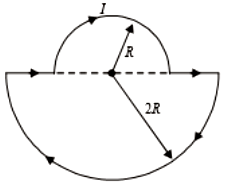1.

2.

3.

4.  $5{\mathrm{\pi R}}^{2}\mathrm{I}$

Concept Questions :-

Magnetic field due to various cases
High Yielding Test Series + Question Bank - NEET 2020

Difficulty Level:

The magnetic field at center due to the orbital motion of the electron in the hydrogen atom is :

1.  12.5 tesla

2.  5 tesla

3.  24 tesla

4.  1 tesla

Concept Questions :-

Magnetic field due to various cases
High Yielding Test Series + Question Bank - NEET 2020

Difficulty Level:

The figure shows a particle of charge q and mass m moving with velocity v along the x-axis enters a region of the uniform magnetic field. The minimum value of v so that the charge q is deflected by an angle $30°$ is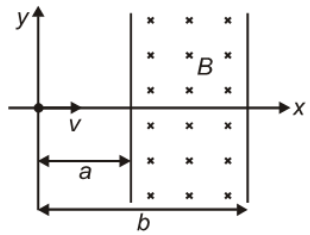1.

2.

3.

4.  $\frac{\mathrm{qBb}}{2\mathrm{m}}$

Concept Questions :-

Lorentz force
High Yielding Test Series + Question Bank - NEET 2020

Difficulty Level:

An electron is traveling along the x-direction. It encounters a magnetic field in the y-direction. Its subsequent motion will be:

1.  Straight-line along the x-direction

2.  A circle in the xz-plane

3.  A circle in the yz-plane

4.  A circle in the xy-plane

Concept Questions :-

Lorentz force